# Program for Gauss-Jordan Elimination Method

Prerequisite : Gaussian Elimination to Solve Linear Equations

Introduction : The Gauss-Jordan method, also known as Gauss-Jordan elimination method is used to solve a system of linear equations and is a modified version of Gauss Elimination Method.

It is similar and simpler than Gauss Elimination Method as we have to perform 2 different process in Gauss Elimination Method i.e.
1) Formation of upper triangular matrix, and
2) Back substitution

But in case of Gauss-Jordan Elimination Method, we only have to form a reduced row echelon form (diagonal matrix). Below given is the flow-chart of Gauss-Jordan Elimination Method.

Flow Chart of Gauss-Jordan Elimination Method :Examples :

```Input :  2y + z = 4
x + y + 2z = 6
2x + y + z = 7

Output :
Final Augumented Matrix is :
1 0 0 2.2
0 2 0 2.8
0 0 -2.5 -3

Result is : 2.2 1.4 1.2
```

Explanation : Below given is the explanation of the above example.

• Input Augmented Matrix is :
•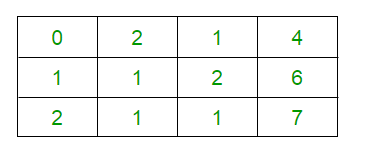• Interchanging R1 and R2, we get
•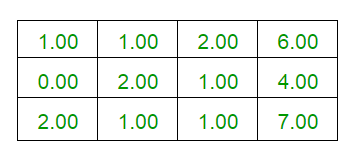• Performing the row operation R3 <- R3 – (2*R1)
•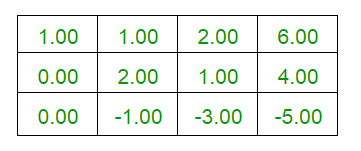• Performing the row operations R1 <- R1 – ((1/2)* R2) and R3 <- R3 + ((1/2)*R2)
•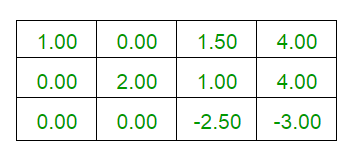• Performing R1 <- R1 + ((3/5)*R3) and R2 <- R2 + ((2/5)*R3)
•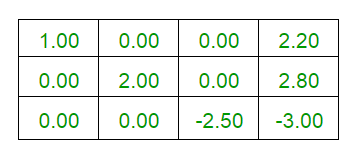• Unique Solutions are :
•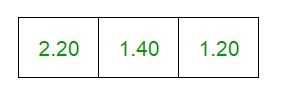## C++

 `// C++ Implementation for Gauss-Jordan ` `// Elimination Method ` `#include ` `using` `namespace` `std; ` ` `  `#define M 10 ` ` `  `// Function to print the matrix ` `void` `PrintMatrix(``float` `a[][M], ``int` `n) ` `{ ` `    ``for` `(``int` `i = 0; i < n; i++) { ` `        ``for` `(``int` `j = 0; j <= n; j++)  ` `          ``cout << a[i][j] << ``" "``; ` `        ``cout << endl; ` `    ``} ` `} ` ` `  `// function to reduce matrix to reduced ` `// row echelon form. ` `int` `PerformOperation(``float` `a[][M], ``int` `n) ` `{ ` `    ``int` `i, j, k = 0, c, flag = 0, m = 0; ` `    ``float` `pro = 0; ` `     `  `    ``// Performing elementary operations ` `    ``for` `(i = 0; i < n; i++) ` `    ``{ ` `        ``if` `(a[i][i] == 0)  ` `        ``{ ` `            ``c = 1; ` `            ``while` `((i + c) < n && a[i + c][i] == 0)  ` `                ``c++;             ` `            ``if` `((i + c) == n) { ` `                ``flag = 1; ` `                ``break``; ` `            ``} ` `            ``for` `(j = i, k = 0; k <= n; k++)  ` `                ``swap(a[j][k], a[j+c][k]); ` `        ``} ` ` `  `        ``for` `(j = 0; j < n; j++) { ` `             `  `            ``// Excluding all i == j ` `            ``if` `(i != j) { ` `                 `  `                ``// Converting Matrix to reduced row ` `                ``// echelon form(diagonal matrix) ` `                ``float` `pro = a[j][i] / a[i][i]; ` ` `  `                ``for` `(k = 0; k <= n; k++)                  ` `                    ``a[j][k] = a[j][k] - (a[i][k]) * pro;                 ` `            ``} ` `        ``} ` `    ``} ` `    ``return` `flag; ` `} ` ` `  `// Function to print the desired result  ` `// if unique solutions exists, otherwise  ` `// prints no solution or infinite solutions  ` `// depending upon the input given. ` `void` `PrintResult(``float` `a[][M], ``int` `n, ``int` `flag) ` `{ ` `    ``cout << ``"Result is : "``; ` ` `  `    ``if` `(flag == 2)      ` `      ``cout << ``"Infinite Solutions Exists"` `<< endl;     ` `    ``else` `if` `(flag == 3)      ` `      ``cout << ``"No Solution Exists"` `<< endl; ` `     `  `     `  `    ``// Printing the solution by dividing constants by ` `    ``// their respective diagonal elements ` `    ``else` `{ ` `        ``for` `(``int` `i = 0; i < n; i++)          ` `            ``cout << a[i][n] / a[i][i] << ``" "``;         ` `    ``} ` `} ` ` `  `// To check whether infinite solutions  ` `// exists or no solution exists ` `int` `CheckConsistency(``float` `a[][M], ``int` `n, ``int` `flag) ` `{ ` `    ``int` `i, j; ` `    ``float` `sum; ` `     `  `    ``// flag == 2 for infinite solution ` `    ``// flag == 3 for No solution ` `    ``flag = 3; ` `    ``for` `(i = 0; i < n; i++)  ` `    ``{ ` `        ``sum = 0; ` `        ``for` `(j = 0; j < n; j++)         ` `            ``sum = sum + a[i][j]; ` `        ``if` `(sum == a[i][j])  ` `            ``flag = 2;         ` `    ``} ` `    ``return` `flag; ` `} ` ` `  `// Driver code ` `int` `main() ` `{ ` `    ``float` `a[M][M] = {{ 0, 2, 1, 4 },  ` `                     ``{ 1, 1, 2, 6 },  ` `                     ``{ 2, 1, 1, 7 }}; ` `                      `  `    ``// Order of Matrix(n) ` `    ``int` `n = 3, flag = 0; ` `     `  `    ``// Performing Matrix transformation ` `    ``flag = PerformOperation(a, n); ` `     `  `    ``if` `(flag == 1)      ` `        ``flag = CheckConsistency(a, n, flag);     ` ` `  `    ``// Printing Final Matrix ` `    ``cout << ``"Final Augumented Matrix is : "` `<< endl; ` `    ``PrintMatrix(a, n); ` `    ``cout << endl; ` `     `  `    ``// Printing Solutions(if exist) ` `    ``PrintResult(a, n, flag); ` ` `  `    ``return` `0; ` `} `

## Java

 `// Java Implementation for Gauss-Jordan ` `// Elimination Method ` `class` `GFG { ` `     `  `static` `int` `M = ``10``; ` ` `  `// Function to print the matrix ` `static` `void` `PrintMatrix(``float` `a[][], ``int` `n) ` `{ ` `    ``for` `(``int` `i = ``0``; i < n; i++)  ` `    ``{ ` `        ``for` `(``int` `j = ``0``; j <= n; j++)  ` `            ``System.out.print(a[i][j] + ``" "``); ` `        ``System.out.println(); ` `    ``} ` `} ` ` `  `// function to reduce matrix to reduced ` `// row echelon form. ` `static` `int` `PerformOperation(``float` `a[][], ``int` `n) ` `{ ` `    ``int` `i, j, k = ``0``, c, flag = ``0``, m = ``0``; ` `    ``float` `pro = ``0``; ` `     `  `    ``// Performing elementary operations ` `    ``for` `(i = ``0``; i < n; i++) ` `    ``{ ` `        ``if` `(a[i][i] == ``0``)  ` `        ``{ ` `            ``c = ``1``; ` `            ``while` `((i + c) < n && a[i + c][i] == ``0``)  ` `                ``c++;          ` `            ``if` `((i + c) == n)  ` `            ``{ ` `                ``flag = ``1``; ` `                ``break``; ` `            ``} ` `            ``for` `(j = i, k = ``0``; k <= n; k++)  ` `            ``{ ` `                ``float` `temp =a[j][k]; ` `                ``a[j][k] = a[j+c][k]; ` `                ``a[j+c][k] = temp; ` `            ``} ` `        ``} ` ` `  `        ``for` `(j = ``0``; j < n; j++)  ` `        ``{ ` `             `  `            ``// Excluding all i == j ` `            ``if` `(i != j)  ` `            ``{ ` `                 `  `                ``// Converting Matrix to reduced row ` `                ``// echelon form(diagonal matrix) ` `                ``float` `p = a[j][i] / a[i][i]; ` ` `  `                ``for` `(k = ``0``; k <= n; k++)                  ` `                    ``a[j][k] = a[j][k] - (a[i][k]) * p;              ` `            ``} ` `        ``} ` `    ``} ` `    ``return` `flag; ` `} ` ` `  `// Function to print the desired result  ` `// if unique solutions exists, otherwise  ` `// prints no solution or infinite solutions  ` `// depending upon the input given. ` `static` `void` `PrintResult(``float` `a[][], ``int` `n, ``int` `flag) ` `{ ` `    ``System.out.print(``"Result is : "``); ` ` `  `    ``if` `(flag == ``2``)      ` `    ``System.out.println(``"Infinite Solutions Exists"``);  ` `    ``else` `if` `(flag == ``3``)      ` `    ``System.out.println(``"No Solution Exists"``); ` `     `  `     `  `    ``// Printing the solution by dividing constants by ` `    ``// their respective diagonal elements ` `    ``else` `{ ` `        ``for` `(``int` `i = ``0``; i < n; i++)          ` `            ``System.out.print(a[i][n] / a[i][i] +``" "``);      ` `    ``} ` `} ` ` `  `// To check whether infinite solutions  ` `// exists or no solution exists ` `static` `int` `CheckConsistency(``float` `a[][], ``int` `n, ``int` `flag) ` `{ ` `    ``int` `i, j; ` `    ``float` `sum; ` `     `  `    ``// flag == 2 for infinite solution ` `    ``// flag == 3 for No solution ` `    ``flag = ``3``; ` `    ``for` `(i = ``0``; i < n; i++)  ` `    ``{ ` `        ``sum = ``0``; ` `        ``for` `(j = ``0``; j < n; j++)      ` `            ``sum = sum + a[i][j]; ` `        ``if` `(sum == a[i][j])  ` `            ``flag = ``2``;      ` `    ``} ` `    ``return` `flag; ` `} ` ` `  `// Driver code ` `public` `static` `void` `main(String[] args)  ` `{ ` `    ``float` `a[][] = {{ ``0``, ``2``, ``1``, ``4` `},  ` `                    ``{ ``1``, ``1``, ``2``, ``6` `},  ` `                    ``{ ``2``, ``1``, ``1``, ``7` `}}; ` `                     `  `    ``// Order of Matrix(n) ` `    ``int` `n = ``3``, flag = ``0``; ` `     `  `    ``// Performing Matrix transformation ` `    ``flag = PerformOperation(a, n); ` `     `  `    ``if` `(flag == ``1``)      ` `        ``flag = CheckConsistency(a, n, flag);  ` ` `  `    ``// Printing Final Matrix ` `    ``System.out.println(``"Final Augumented Matrix is : "``); ` `    ``PrintMatrix(a, n); ` `    ``System.out.println(``""``); ` `     `  `    ``// Printing Solutions(if exist) ` `    ``PrintResult(a, n, flag); ` `} ` `} ` ` `  `/* This code contributed by PrinciRaj1992 */`

## C#

 `// C# Implementation for Gauss-Jordan ` `// Elimination Method ` `using` `System; ` `using` `System.Collections.Generic; ` ` `  `class` `GFG ` `{ ` `static` `int` `M = 10; ` ` `  `// Function to print the matrix ` `static` `void` `PrintMatrix(``float` `[,]a, ``int` `n) ` `{ ` `    ``for` `(``int` `i = 0; i < n; i++)  ` `    ``{ ` `        ``for` `(``int` `j = 0; j <= n; j++)  ` `            ``Console.Write(a[i, j] + ``" "``); ` `        ``Console.WriteLine(); ` `    ``} ` `} ` ` `  `// function to reduce matrix to reduced ` `// row echelon form. ` `static` `int` `PerformOperation(``float` `[,]a, ``int` `n) ` `{ ` `    ``int` `i, j, k = 0, c, flag = 0; ` `     `  `    ``// Performing elementary operations ` `    ``for` `(i = 0; i < n; i++) ` `    ``{ ` `        ``if` `(a[i, i] == 0)  ` `        ``{ ` `            ``c = 1; ` `            ``while` `((i + c) < n && a[i + c, i] == 0)  ` `                ``c++;          ` `            ``if` `((i + c) == n)  ` `            ``{ ` `                ``flag = 1; ` `                ``break``; ` `            ``} ` `            ``for` `(j = i, k = 0; k <= n; k++)  ` `            ``{ ` `                ``float` `temp = a[j, k]; ` `                ``a[j, k] = a[j + c, k]; ` `                ``a[j + c, k] = temp; ` `            ``} ` `        ``} ` ` `  `        ``for` `(j = 0; j < n; j++)  ` `        ``{ ` `             `  `            ``// Excluding all i == j ` `            ``if` `(i != j)  ` `            ``{ ` `                 `  `                ``// Converting Matrix to reduced row ` `                ``// echelon form(diagonal matrix) ` `                ``float` `p = a[j, i] / a[i, i]; ` ` `  `                ``for` `(k = 0; k <= n; k++)                  ` `                    ``a[j, k] = a[j, k] - (a[i, k]) * p;              ` `            ``} ` `        ``} ` `    ``} ` `    ``return` `flag; ` `} ` ` `  `// Function to print the desired result  ` `// if unique solutions exists, otherwise  ` `// prints no solution or infinite solutions  ` `// depending upon the input given. ` `static` `void` `PrintResult(``float` `[,]a, ` `                        ``int` `n, ``int` `flag) ` `{ ` `    ``Console.Write(``"Result is : "``); ` ` `  `    ``if` `(flag == 2)      ` `    ``Console.WriteLine(``"Infinite Solutions Exists"``);  ` `    ``else` `if` `(flag == 3)      ` `    ``Console.WriteLine(``"No Solution Exists"``); ` `     `  `    ``// Printing the solution by dividing  ` `    ``// constants by their respective ` `    ``// diagonal elements ` `    ``else`  `    ``{ ` `        ``for` `(``int` `i = 0; i < n; i++)          ` `            ``Console.Write(a[i, n] / a[i, i] + ``" "``);      ` `    ``} ` `} ` ` `  `// To check whether infinite solutions  ` `// exists or no solution exists ` `static` `int` `CheckConsistency(``float` `[,]a,  ` `                            ``int` `n, ``int` `flag) ` `{ ` `    ``int` `i, j; ` `    ``float` `sum; ` `     `  `    ``// flag == 2 for infinite solution ` `    ``// flag == 3 for No solution ` `    ``flag = 3; ` `    ``for` `(i = 0; i < n; i++)  ` `    ``{ ` `        ``sum = 0; ` `        ``for` `(j = 0; j < n; j++)      ` `            ``sum = sum + a[i, j]; ` `        ``if` `(sum == a[i, j])  ` `            ``flag = 2;      ` `    ``} ` `    ``return` `flag; ` `} ` ` `  `// Driver code ` `public` `static` `void` `Main(String[] args)  ` `{ ` `    ``float` `[,]a = {{ 0, 2, 1, 4 },  ` `                  ``{ 1, 1, 2, 6 },  ` `                  ``{ 2, 1, 1, 7 }}; ` `                     `  `    ``// Order of Matrix(n) ` `    ``int` `n = 3, flag = 0; ` `     `  `    ``// Performing Matrix transformation ` `    ``flag = PerformOperation(a, n); ` `     `  `    ``if` `(flag == 1)      ` `        ``flag = CheckConsistency(a, n, flag);  ` ` `  `    ``// Printing Final Matrix ` `    ``Console.WriteLine(``"Final Augumented Matrix is : "``); ` `    ``PrintMatrix(a, n); ` `    ``Console.WriteLine(``""``); ` `     `  `    ``// Printing Solutions(if exist) ` `    ``PrintResult(a, n, flag); ` `} ` `} ` ` `  `// This code is contributed by 29AjayKumar `

Output:

```Final Augumented Matrix is :
1 0 0 2.2
0 2 0 2.8
0 0 -2.5 -3

Result is : 2.2 1.4 1.2
```

Applications :

• Solving System of Linear Equations : Gauss-Jordan Elimination Method can be used for finding the solution of a systems of linear equations which is applied throughout the mathematics.
• Finding Determinant : The Gaussian Elimination can be applied to a square matrix in order to find determinant of the matrix.
• Finding Inverse of Matrix : The Gauss-Jordan Elimination method can be used in determining the inverse of a square matrix.
• Finding Ranks and Bases : Using reduced row echelon form, the ranks as well as bases of square matrices can be computed by Gaussian elimination method.

Don’t stop now and take your learning to the next level. Learn all the important concepts of Data Structures and Algorithms with the help of the most trusted course: DSA Self Paced. Become industry ready at a student-friendly price.

My Personal Notes arrow_drop_upCheck out this Author's contributed articles.

If you like GeeksforGeeks and would like to contribute, you can also write an article using contribute.geeksforgeeks.org or mail your article to contribute@geeksforgeeks.org. See your article appearing on the GeeksforGeeks main page and help other Geeks.

Please Improve this article if you find anything incorrect by clicking on the "Improve Article" button below.

Article Tags :
Practice Tags :

1

Please write to us at contribute@geeksforgeeks.org to report any issue with the above content.